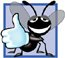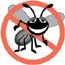# Another C++ Program: Adding Integers

### Another C++ Program Adding Integers

Our next program uses the input stream object std::cin and the stream extraction operator, >>, to obtain two integers typed by a user at the keyboard, computes the sum of these values and outputs the result using std::cout. Figure 2.5 shows the program and sample inputs and outputs. Note that we highlight the user's input in bold.

Figure 2.5. Addition program that displays the sum of two integers entered at the keyboard.

(This item is displayed on page 43 in the print version)

``` 1 // Fig. 2.5: fig02_05.cpp
2 // Addition program that displays the sum of two numbers.
3 #include  // allows program to perform input and output
4
5 // function main begins program execution
6 int main()
7 {
8 // variable declarations
9 int number1; // first integer to add
10 int number2; // second integer to add
11 int sum; // sum of number1 and number2
12
13 std::cout << "Enter first integer: "; // prompt user for data
14 std::cin >> number1; // read first integer from user into number1
15
16 std::cout << "Enter second integer: "; // prompt user for data
17 std::cin >> number2; // read second integer from user into number2
18
19 sum = number1 + number2; // add the numbers; store result in sum
20
21 std::cout << "Sum is " << sum << std::end1; // display sum; end line
22
23 return 0; // indicate that program ended successfully
24
25 } // end function main
```

 ``` Enter first integer: 45 Enter second integer: 72 Sum is 117 ```

The comments in lines 1 and 2

```// Fig. 2.5: fig02_05.cpp
// Addition program that displays the sum of two numbers.
```

state the name of the file and the purpose of the program. The C++ preprocessor directive

```#include  // allows program to perform input and output
```

in line 3 includes the contents of the iostream header file in the program.

The program begins execution with function main (line 6). The left brace (line 7) marks the beginning of main's body and the corresponding right brace (line 25) marks the end of main.

Lines 911

```int number1; // first integer to add
int number2; // second integer to add
int sum; // sum of number1 and number2
```

are declarations. The identifiers number1, number2 and sum are the names of variables. A variable is a location in the computer's memory where a value can be stored for use by a program. These declarations specify that the variables number1, number2 and sum are data of type int, meaning that these variables will hold integer values, i.e., whole numbers such as 7, 11, 0 and 31914. All variables must be declared with a name and a data type before they can be used in a program. Several variables of the same type may be declared in one declaration or in multiple declarations. We could have declared all three variables in one declaration as follows:

```int number1, number2, sum;
```

This makes the program less readable and prevents us from providing comments that describe each variable's purpose. If more than one name is declared in a declaration (as shown here), the names are separated by commas (,). This is referred to as a comma-separated list.

Good Programming Practice 2.6Place a space after each comma (,) to make programs more readable.

Good Programming Practice 2.7Some programmers prefer to declare each variable on a separate line. This format allows for easy insertion of a descriptive comment next to each declaration.

We will soon discuss the data type double for specifying real numbers, and the data type char for specifying character data. Real numbers are numbers with decimal points, such as 3.4, 0.0 and 11.19. A char variable may hold only a single lowercase letter, a single uppercase letter, a single digit or a single special character (e.g., \$ or *). Types such as int, double and char are often called fundamental types, primitive types or built-in types. Fundamental-type names are keywords and therefore must appear in all lowercase letters. Appendix C contains the complete list of fundamental types.

A variable name (such as number1) is any valid identifier that is not a keyword. An identifier is a series of characters consisting of letters, digits and underscores (_) that does not begin with a digit. C++ is case sensitiveuppercase and lowercase letters are different, so a1 and A1 are different identifiers.

Portability Tip 2.1C++ allows identifiers of any length, but your C++ implementation may impose some restrictions on the length of identifiers. Use identifiers of 31 characters or fewer to ensure portability.

Good Programming Practice 2.8Choosing meaningful identifiers helps make a program self-documentinga person can understand the program simply by reading it rather than having to refer to manuals or comments.

Good Programming Practice 2.9Avoid using abbreviations in identifiers. This promotes program readability.

Good Programming Practice 2.10Avoid identifiers that begin with underscores and double underscores, because C++ compilers may use names like that for their own purposes internally. This will prevent names you choose from being confused with names the compilers choose.

Error-Prevention Tip 2.1Languages like C++ are "moving targets." As they evolve, more keywords could be added to the language. Avoid using "loaded" words like "object" as identifiers. Even though "object" is not currently a keyword in C++, it could become one; therefore, future compiling with new compilers could break existing code.

Declarations of variables can be placed almost anywhere in a program, but they must appear before their corresponding variables are used in the program. For example, in the program of Fig. 2.5, the declaration in line 9

```int number1; // first integer to add
```

could have been placed immediately before line 14

```std::cin >> number1; // read first integer from user into number1
```

the declaration in line 10

```int number2; // second integer to add
```

could have been placed immediately before line 17

```std::cin >> number2; // read second integer from user into number2
```

and the declaration in line 11

```int sum; // sum of number1 and number2
```

could have been placed immediately before line 19

```sum = number1 + number2; // add the numbers; store result in sum
```

Good Programming Practice 2.11Always place a blank line between a declaration and adjacent executable statements. This makes the declarations stand out in the program and contributes to program clarity.

Good Programming Practice 2.12If you prefer to place declarations at the beginning of a function, separate them from the executable statements in that function with one blank line to highlight where the declarations end and the executable statements begin.

Line 13

```std::cout << "Enter first integer: "; // prompt user for data
```

prints the string Enter first integer: (also known as a string literal or a literal) on the screen. This message is called a prompt because it directs the user to take a specific action. We like to pronounce the preceding statement as "std::cout gets the character string "Enter first integer: "." Line 14

```std::cin >> number1; // read first integer from user into number1
```

uses the input stream object cin (of namespace std) and the stream extraction operator, >>, to obtain a value from the keyboard. Using the stream extraction operator with std::cin takes character input from the standard input stream, which is usually the keyboard. We like to pronounce the preceding statement as, "std::cin gives a value to number1" or simply "std::cin gives number1."

Error-Prevention Tip 2.2Programs should validate the correctness of all input values to prevent erroneous information from affecting a program's calculations.

When the computer executes the preceding statement, it waits for the user to enter a value for variable number1. The user responds by typing an integer (as characters), then pressing the Enter key (sometimes called the Return key) to send the characters to the computer. The computer converts the character representation of the number to an integer and assigns (copies) this number (or value) to the variable number1. Any subsequent references to number1 in this program will use this same value.

The std::cout and std::cin stream objects facilitate interaction between the user and the computer. Because this interaction resembles a dialog, it is often called conversational computing or interactive computing.

Line 16

```std::cout << "Enter second integer: "; // prompt user for data
```

prints Enter second integer: on the screen, prompting the user to take action. Line 17

```std::cin >> number2; // read second integer from user into number2
```

obtains a value for variable number2 from the user.

The assignment statement in line 19

```sum = number1 + number2; // add the numbers; store result in sum
```

calculates the sum of the variables number1 and number2 and assigns the result to variable sum using the assignment operator =. The statement is read as, "sum gets the value of number1 + number2." Most calculations are performed in assignment statements. The = operator and the + operator are called binary operators because each has two operands. In the case of the + operator, the two operands are number1 and number2. In the case of the preceding = operator, the two operands are sum and the value of the expression number1 + number2.

Good Programming Practice 2.13Place spaces on either side of a binary operator. This makes the operator stand out and makes the program more readable.

Line 21

```std::cout << "Sum is " << sum << std::endl; // display sum; end line
```

displays the character string Sum is followed by the numerical value of variable sum followed by std::endla so-called stream manipulator. The name endl is an abbreviation for "end line" and belongs to namespace std. The std::endl stream manipulator outputs a newline, then "flushes the output buffer." This simply means that, on some systems where outputs accumulate in the machine until there are enough to "make it worthwhile" to display on the screen, std::endl forces any accumulated outputs to be displayed at that moment. This can be important when the outputs are prompting the user for an action, such as entering data.

Note that the preceding statement outputs multiple values of different types. The stream insertion operator "knows" how to output each type of data. Using multiple stream insertion operators (<<) in a single statement is referred to as concatenating, chaining or cascading stream insertion operations. It is unnecessary to have multiple statements to output multiple pieces of data.

Calculations can also be performed in output statements. We could have combined the statements in lines 19 and 21 into the statement

```std::cout << "Sum is " << number1 + number2 << std::endl;
```

thus eliminating the need for the variable sum.

A powerful feature of C++ is that users can create their own data types called classes (we introduce this capability in Chapter 3 and explore it in depth in Chapters 9 and 10). Users can then "teach" C++ how to input and output values of these new data types using the >> and << operators (this is called operator overloadinga topic we explore in Chapter 11).C++ How to Program (5th Edition)
ISBN: 0131857576
EAN: 2147483647
Year: 2004
Pages: 627